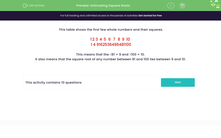# Estimate the Square Roots of Numbers

In this worksheet, students will estimate square roots.Key stage:  KS 3

Curriculum topic:   Number

Curriculum subtopic:   Understand Integer Powers/Real Roots

Popular topics:   Estimation worksheets

Difficulty level:#### Worksheet Overview

This table shows the first few whole numbers and their squares.

 1 2 3 4 5 6 7 8 9 10 1 4 9 16 25 36 49 64 81 100

From the table, we can see that the √81 = 9 and √100 = 10.

It also means that we can find an estimate for the cube root of any number between 81 and 100.

For example, the √89 lies between 9 and 10.

In this activity, we are going to use known square roots to estimate the square roots of more complicated numbers.

Let's get started.

### What is EdPlace?

We're your National Curriculum aligned online education content provider helping each child succeed in English, maths and science from year 1 to GCSE. With an EdPlace account you’ll be able to track and measure progress, helping each child achieve their best. We build confidence and attainment by personalising each child’s learning at a level that suits them.

Get started